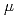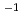The equivalent dose rate per positron fluence for the human eye lens is calculated using a Monte Carlo simulation code, EGS5, with two mathematical models (a computational head voxel phantom and a simple eye model). The eye lens doses for medical staff and carers as received from a radiopharmaceutical such as 18F-FDG, which is used in PET examinations, when spilled on the floor, and from positrons passing through a thin injection tube are calculated using a simple eye model. At energies less than 1 MeV, the equivalent dose for the eye lens per electron and positron fluence as calculated using the simple eye model was a maximum of three orders of magnitude lower than that for the head voxel phantom. These values for the simple eye model were equal to the calculation results obtained using the eye lens model in ICRP Publication 116 and those reported in past studies. The eye lens dose rates from the point and tube sources of 18F-FDG as calculated using our simple eye model are about 0.57 and 0.37Sv minMBqat 5 cm, respectively. The doses at 10 cm were one-third to half of those at 5 cm.